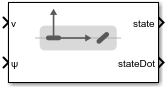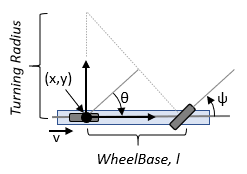# Bicycle Kinematic Model

Compute car-like vehicle motion using bicycle kinematic model

Since R2019b

•Libraries:
Robotics System Toolbox / Mobile Robot Algorithms

## Description

The Bicycle Kinematic Model block creates a bicycle vehicle model to simulate simplified car-like vehicle dynamics. This model represents a vehicle with two axles defined by the length between the axles, `Wheel base`. The front wheel can be turned with steering angle `psi`. The vehicle heading `theta` is defined at the center of the rear axle.## Ports

### Input

expand all

Vehicle speed, specified in meters per second.

Steering angle of the vehicle, specified in radians.

#### Dependencies

To enable this port, set the ```Vehicle inputs``` parameter to ```Vehicle Speed & Steering Angle```.

Angular velocity of the vehicle, specified in radians per second. A positive value steers the vehicle left and negative values steer the vehicle right.

#### Dependencies

To enable this port, set the ```Vehicle inputs``` parameter to ```Vehicle Speed & Heading Angular Velocity```.

### Output

expand all

Current xy-position and orientation of the vehicle, specified as a [x y theta] vector in meters and radians.

The linear and angular velocities of the vehicle, specified as a [xDot yDot thetaDot] vector in meters per second and radians per second. The linear and angular velocities are calculated by taking the derivative of the `state` output.

## Parameters

expand all

Type of speed and directional inputs for vehicle, specified as one of these options:

• `Vehicle Speed & Steering Angle` — Vehicle speed in meters per second with a steering angle in radians.

• `Vehicle Speed & Heading Angular Velocity` — Vehicle speed in meters per second with a heading angular velocity in radians per second.

The wheel base refers to the distance between the front and rear vehicle axles, specified in meters.

The wheel speed range is a two-element vector that provides the minimum and maximum vehicle wheel speeds, [MinSpeed MaxSpeed], specified in radians per second.

The maximum steering angle, refers to the maximum amount the vehicle can be steered to the right or left, specified in radians. This property is used to validate the user-provided state input.

If `Maximum steering angle` is set to `pi/2`, it results in a minimum turning radius of zero meters.

The initial x-, `y`-position and orientation, theta, of the vehicle.

• `Interpreted execution` — Simulate model using the MATLAB® interpreter. For more information, see Simulation Modes (Simulink).

• `Code generation` — Simulate model using generated C code. The first time you run a simulation, Simulink® generates C code for the block. The C code is reused for subsequent simulations, as long as the model does not change.

Tunable: No

 Lynch, Kevin M., and Frank C. Park. Modern Robotics: Mechanics, Planning, and Control 1st ed. Cambridge, MA: Cambridge University Press, 2017.

 Corke, Peter I. Robotics, Vision and Control: Fundamental Algorithms in MATLAB. Springer, 2011.